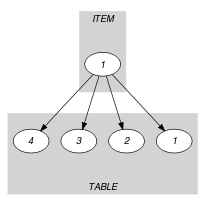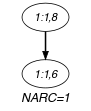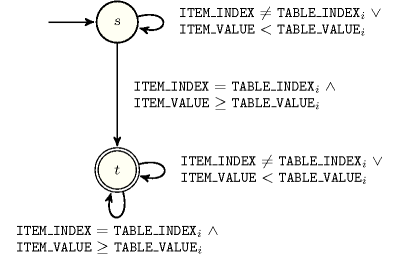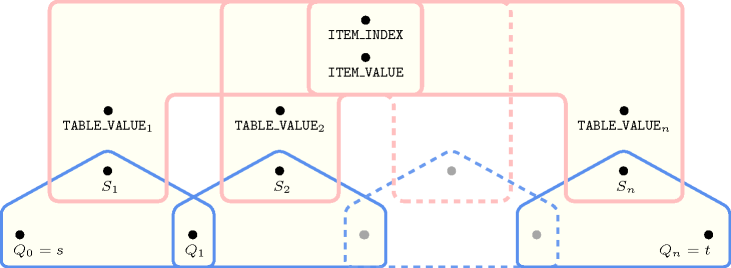## 5.140. element_greatereq

Origin
Constraint

$\mathrm{𝚎𝚕𝚎𝚖𝚎𝚗𝚝}_\mathrm{𝚐𝚛𝚎𝚊𝚝𝚎𝚛𝚎𝚚}\left(\mathrm{𝙸𝚃𝙴𝙼},\mathrm{𝚃𝙰𝙱𝙻𝙴}\right)$

Arguments
 $\mathrm{𝙸𝚃𝙴𝙼}$ $\mathrm{𝚌𝚘𝚕𝚕𝚎𝚌𝚝𝚒𝚘𝚗}\left(\mathrm{𝚒𝚗𝚍𝚎𝚡}-\mathrm{𝚍𝚟𝚊𝚛},\mathrm{𝚟𝚊𝚕𝚞𝚎}-\mathrm{𝚍𝚟𝚊𝚛}\right)$ $\mathrm{𝚃𝙰𝙱𝙻𝙴}$ $\mathrm{𝚌𝚘𝚕𝚕𝚎𝚌𝚝𝚒𝚘𝚗}\left(\mathrm{𝚒𝚗𝚍𝚎𝚡}-\mathrm{𝚒𝚗𝚝},\mathrm{𝚟𝚊𝚕𝚞𝚎}-\mathrm{𝚒𝚗𝚝}\right)$
Restrictions
 $\mathrm{𝚛𝚎𝚚𝚞𝚒𝚛𝚎𝚍}$$\left(\mathrm{𝙸𝚃𝙴𝙼},\left[\mathrm{𝚒𝚗𝚍𝚎𝚡},\mathrm{𝚟𝚊𝚕𝚞𝚎}\right]\right)$ $\mathrm{𝙸𝚃𝙴𝙼}.\mathrm{𝚒𝚗𝚍𝚎𝚡}\ge 1$ $\mathrm{𝙸𝚃𝙴𝙼}.\mathrm{𝚒𝚗𝚍𝚎𝚡}\le |\mathrm{𝚃𝙰𝙱𝙻𝙴}|$ $|\mathrm{𝙸𝚃𝙴𝙼}|=1$ $|\mathrm{𝚃𝙰𝙱𝙻𝙴}|>0$ $\mathrm{𝚛𝚎𝚚𝚞𝚒𝚛𝚎𝚍}$$\left(\mathrm{𝚃𝙰𝙱𝙻𝙴},\left[\mathrm{𝚒𝚗𝚍𝚎𝚡},\mathrm{𝚟𝚊𝚕𝚞𝚎}\right]\right)$ $\mathrm{𝚃𝙰𝙱𝙻𝙴}.\mathrm{𝚒𝚗𝚍𝚎𝚡}\ge 1$ $\mathrm{𝚃𝙰𝙱𝙻𝙴}.\mathrm{𝚒𝚗𝚍𝚎𝚡}\le |\mathrm{𝚃𝙰𝙱𝙻𝙴}|$ $\mathrm{𝚍𝚒𝚜𝚝𝚒𝚗𝚌𝚝}$$\left(\mathrm{𝚃𝙰𝙱𝙻𝙴},\mathrm{𝚒𝚗𝚍𝚎𝚡}\right)$
Purpose

$\mathrm{𝙸𝚃𝙴𝙼}\left[1\right].\mathrm{𝚟𝚊𝚕𝚞𝚎}$ is greater than or equal to one of the entries (i.e., the $\mathrm{𝚟𝚊𝚕𝚞𝚎}$ attribute) of the table $\mathrm{𝚃𝙰𝙱𝙻𝙴}$.

Example
$\left(\begin{array}{c}〈\mathrm{𝚒𝚗𝚍𝚎𝚡}-1\mathrm{𝚟𝚊𝚕𝚞𝚎}-8〉,\hfill \\ 〈\begin{array}{cc}\mathrm{𝚒𝚗𝚍𝚎𝚡}-1\hfill & \mathrm{𝚟𝚊𝚕𝚞𝚎}-6,\hfill \\ \mathrm{𝚒𝚗𝚍𝚎𝚡}-2\hfill & \mathrm{𝚟𝚊𝚕𝚞𝚎}-9,\hfill \\ \mathrm{𝚒𝚗𝚍𝚎𝚡}-3\hfill & \mathrm{𝚟𝚊𝚕𝚞𝚎}-2,\hfill \\ \mathrm{𝚒𝚗𝚍𝚎𝚡}-4\hfill & \mathrm{𝚟𝚊𝚕𝚞𝚎}-9\hfill \end{array}〉\hfill \end{array}\right)$

The $\mathrm{𝚎𝚕𝚎𝚖𝚎𝚗𝚝}_\mathrm{𝚐𝚛𝚎𝚊𝚝𝚎𝚛𝚎𝚚}$ constraint holds since $\mathrm{𝙸𝚃𝙴𝙼}\left[1\right].\mathrm{𝚟𝚊𝚕𝚞𝚎}=8$ is greater than or equal to $\mathrm{𝚃𝙰𝙱𝙻𝙴}\left[\mathrm{𝙸𝚃𝙴𝙼}\left[1\right].\mathrm{𝚒𝚗𝚍𝚎𝚡}\right].\mathrm{𝚟𝚊𝚕𝚞𝚎}=\mathrm{𝚃𝙰𝙱𝙻𝙴}\left[1\right].\mathrm{𝚟𝚊𝚕𝚞𝚎}=6$.

Typical
 $|\mathrm{𝚃𝙰𝙱𝙻𝙴}|>1$ $\mathrm{𝚛𝚊𝚗𝚐𝚎}$$\left(\mathrm{𝚃𝙰𝙱𝙻𝙴}.\mathrm{𝚟𝚊𝚕𝚞𝚎}\right)>1$
Symmetries
• Items of $\mathrm{𝚃𝙰𝙱𝙻𝙴}$ are permutable.

• All occurrences of two distinct values in $\mathrm{𝙸𝚃𝙴𝙼}.\mathrm{𝚟𝚊𝚕𝚞𝚎}$ or $\mathrm{𝚃𝙰𝙱𝙻𝙴}.\mathrm{𝚟𝚊𝚕𝚞𝚎}$ can be swapped; all occurrences of a value in $\mathrm{𝙸𝚃𝙴𝙼}.\mathrm{𝚟𝚊𝚕𝚞𝚎}$ or $\mathrm{𝚃𝙰𝙱𝙻𝙴}.\mathrm{𝚟𝚊𝚕𝚞𝚎}$ can be renamed to any unused value.

Usage

Used for modelling variable subscripts in linear constraints [OttossonThorsteinssonHooker99].

Reformulation

By introducing an extra variable $\mathrm{𝚅𝙰𝙻}$, the $\mathrm{𝚎𝚕𝚎𝚖𝚎𝚗𝚝}_\mathrm{𝚐𝚛𝚎𝚊𝚝𝚎𝚛𝚎𝚚}$$\left(〈\mathrm{𝚒𝚗𝚍𝚎𝚡}-\mathrm{𝙸𝙽𝙳𝙴𝚇}\mathrm{𝚟𝚊𝚕𝚞𝚎}-\mathrm{𝚅𝙰𝙻𝚄𝙴}〉,\mathrm{𝚃𝙰𝙱𝙻𝙴}\right)$ constraint can be expressed in term of an $\mathrm{𝚎𝚕𝚎𝚖}$$\left(〈\mathrm{𝚒𝚗𝚍𝚎𝚡}-\mathrm{𝙸𝙽𝙳𝙴𝚇}\mathrm{𝚟𝚊𝚕𝚞𝚎}-\mathrm{𝚅𝙰𝙻}〉,\mathrm{𝚃𝙰𝙱𝙻𝙴}\right)$ constraint and of an inequality constraint $\mathrm{𝚅𝙰𝙻𝚄𝙴}\ge \mathrm{𝚅𝙰𝙻}$.

Keywords
Arc input(s)

$\mathrm{𝙸𝚃𝙴𝙼}$ $\mathrm{𝚃𝙰𝙱𝙻𝙴}$

Arc generator
$\mathrm{𝑃𝑅𝑂𝐷𝑈𝐶𝑇}$$↦\mathrm{𝚌𝚘𝚕𝚕𝚎𝚌𝚝𝚒𝚘𝚗}\left(\mathrm{𝚒𝚝𝚎𝚖},\mathrm{𝚝𝚊𝚋𝚕𝚎}\right)$

Arc arity
Arc constraint(s)
 $•\mathrm{𝚒𝚝𝚎𝚖}.\mathrm{𝚒𝚗𝚍𝚎𝚡}=\mathrm{𝚝𝚊𝚋𝚕𝚎}.\mathrm{𝚒𝚗𝚍𝚎𝚡}$ $•\mathrm{𝚒𝚝𝚎𝚖}.\mathrm{𝚟𝚊𝚕𝚞𝚎}\ge \mathrm{𝚝𝚊𝚋𝚕𝚎}.\mathrm{𝚟𝚊𝚕𝚞𝚎}$
Graph property(ies)
$\mathrm{𝐍𝐀𝐑𝐂}$$=1$

Graph model

Similar to the $\mathrm{𝚎𝚕𝚎𝚖𝚎𝚗𝚝}$ constraint except that the equality constraint of the second condition of the arc constraint is replaced by a greater than or equal to constraint.

Parts (A) and (B) of Figure 5.140.1 respectively show the initial and final graph associated with the Example slot. Since we use the $\mathrm{𝐍𝐀𝐑𝐂}$ graph property, the unique arc of the final graph is stressed in bold.

##### Figure 5.140.1. Initial and final graph of the $\mathrm{𝚎𝚕𝚎𝚖𝚎𝚗𝚝}_\mathrm{𝚐𝚛𝚎𝚊𝚝𝚎𝚛𝚎𝚚}$ constraint(a) (b)
Signature

Since all the $\mathrm{𝚒𝚗𝚍𝚎𝚡}$ attributes of $\mathrm{𝚃𝙰𝙱𝙻𝙴}$ are distinct and because of the first arc constraint the final graph cannot have more than one arc. Therefore we can rewrite $\mathrm{𝐍𝐀𝐑𝐂}=1$ to $\mathrm{𝐍𝐀𝐑𝐂}\ge 1$ and simplify $\underline{\overline{\mathrm{𝐍𝐀𝐑𝐂}}}$ to $\overline{\mathrm{𝐍𝐀𝐑𝐂}}$.

Automaton

Figure 5.140.2 depicts the automaton associated with the $\mathrm{𝚎𝚕𝚎𝚖𝚎𝚗𝚝}_\mathrm{𝚐𝚛𝚎𝚊𝚝𝚎𝚛𝚎𝚚}$ constraint. Let $\mathrm{𝙸𝙽𝙳𝙴𝚇}$ and $\mathrm{𝚅𝙰𝙻𝚄𝙴}$ respectively be the $\mathrm{𝚒𝚗𝚍𝚎𝚡}$ and the $\mathrm{𝚟𝚊𝚕𝚞𝚎}$ attributes of the unique item of the $\mathrm{𝙸𝚃𝙴𝙼}$ collection. Let ${\mathrm{𝙸𝙽𝙳𝙴𝚇}}_{i}$ and ${\mathrm{𝚅𝙰𝙻𝚄𝙴}}_{i}$ respectively be the $\mathrm{𝚒𝚗𝚍𝚎𝚡}$ and the $\mathrm{𝚟𝚊𝚕𝚞𝚎}$ attributes of the ${i}^{th}$ item of the $\mathrm{𝚃𝙰𝙱𝙻𝙴}$ collection. To each quadruple $\left(\mathrm{𝙸𝙽𝙳𝙴𝚇},\mathrm{𝚅𝙰𝙻𝚄𝙴},{\mathrm{𝙸𝙽𝙳𝙴𝚇}}_{i},{\mathrm{𝚅𝙰𝙻𝚄𝙴}}_{i}\right)$ corresponds a 0-1 signature variable ${S}_{i}$ as well as the following signature constraint: $\left(\left(\mathrm{𝙸𝙽𝙳𝙴𝚇}={\mathrm{𝙸𝙽𝙳𝙴𝚇}}_{i}\right)\wedge \left(\mathrm{𝚅𝙰𝙻𝚄𝙴}\ge {\mathrm{𝚅𝙰𝙻𝚄𝙴}}_{i}\right)\right)⇔{S}_{i}$.

##### Figure 5.140.2. Automaton of the $\mathrm{𝚎𝚕𝚎𝚖𝚎𝚗𝚝}_\mathrm{𝚐𝚛𝚎𝚊𝚝𝚎𝚛𝚎𝚚}$ constraint##### Figure 5.140.3. Hypergraph of the reformulation corresponding to the automaton of the $\mathrm{𝚎𝚕𝚎𝚖𝚎𝚗𝚝}_\mathrm{𝚐𝚛𝚎𝚊𝚝𝚎𝚛𝚎𝚚}$ constraint